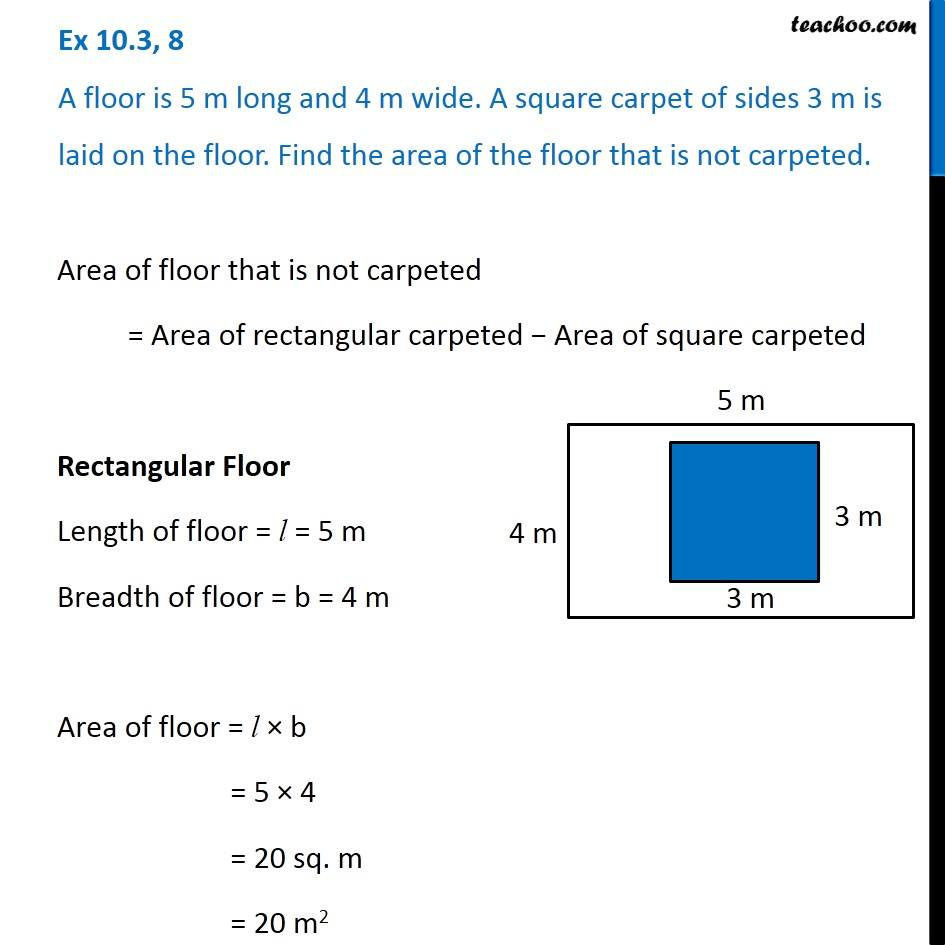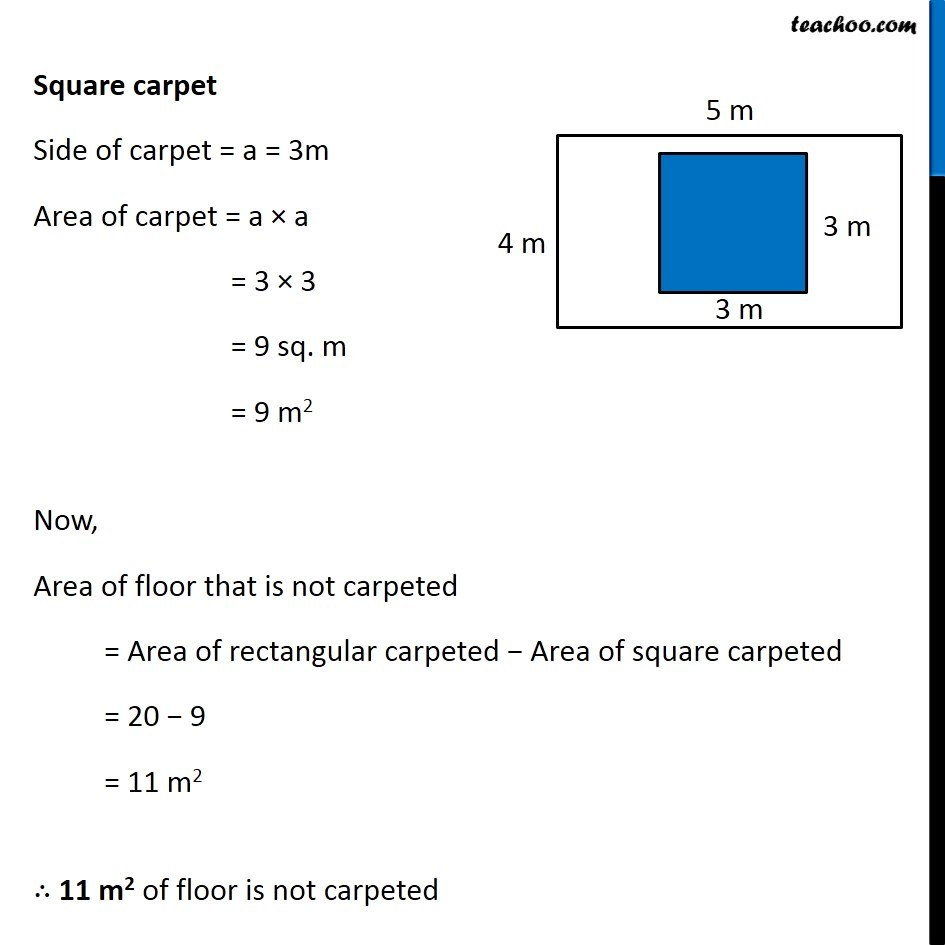Ex 10.3

Chapter 10 Class 6 Mensuration
Serial order wiseGet live Maths 1-on-1 Classs - Class 6 to 12

### Transcript

Ex 10.3, 8 A floor is 5 m long and 4 m wide. A square carpet of sides 3 m is laid on the floor. Find the area of the floor that is not carpeted. Area of floor that is not carpeted = Area of rectangular carpeted − Area of square carpeted Rectangular Floor Length of floor = l = 5 m Breadth of floor = b = 4 m Area of floor = l × b = 5 × 4 = 20 sq. m = 20 m2 Square carpet Side of carpet = a = 3m Area of carpet = a × a = 3 × 3 = 9 sq. m = 9 m2 Now, Area of floor that is not carpeted = Area of rectangular carpeted − Area of square carpeted = 20 − 9 = 11 m2 ∴ 11 m2 of floor is not carpeted